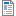# Scratch Math Problem Ideas

I'm interested in collecting Scratch math problems for students to solve. Here's a start--please add more through comments.

I'm interested in collecting Scratch math problems for students to solve. Here's a start--please add more through comments.

1.  Topic: Coordinate Geometry
Project:  Draw the x and y-axes using only “go to x, y” and penup/down blocks.  Extra challenge—draw a coordinate grid as well.

2.  Topic:  Coordinate Geometry, Ordered Pairs, Quadrants
Project:  Draw your initials in quadrant 1 using “go to x, y” and penup/down blocks.  Then draw them in each of the other coordinates, with the same spacing from the x and y-axes.
Comment:  This was harder than I thought.  The kids couldn’t just add negative signs for the other quadrants, they had to figure out new starting positions.  Next time, I would have them draw it out on graph paper first and label some key points to look for strategies in solving the problem.

3.  Topic: Geometry Terms
Project:  Make a Scratch geometry dictionary.  Include at least four terms, with their definitions in your own words plus an animation.  Design an interface so the user can select a term to view.

4.  Topic: Transformations
Project: Make a script that draws a simple cartoon face based on a geometric shape, using “go to x, y” and penup/down blocks.  Make a second script that make an image based on this original and illustrates translation, rotation, reflection across y, or reflection across x.  Add buttons that clear the screen, draw the original, and draw the image.
Comment:  It helps to have a background coordinate grid or to build this on top of the first “draw a grid” project.

5.  Topic: Similarity and Scale Factor
Project: Make a script that draws a simple cartoon face based on a geometric shape, using “go to x, y” and penup/down blocks.  Copy the script and multiply the x coordinate by a scale factor, making a variable “scalefactorx.”  Have the variable value determined by a slider. Then do the same for the y coordinates.  Explore the images you can create.  When are they similar to the original?

6.  Topic:  Probability
Project:  Create a project that uses random numbers to generate a probability experiment, for example flipping a coin or rolling a dice.  Run several tests and give your experimental probability, then compare your results to the theoretical probability of each outcome.

7.  Topic:  Linear Equations, Slope and Intercept
Demonstration:  See Dr. Super’s project http://scratch.mit.edu/projects/DrSuper/323304

Other Files:Scratch Pre-algebra Project Ideas.doc